In :
# This is always required for inline plot rendering in IPython Notebooks; might
# as well do it first, even before the markdown sections, just to be safe
%matplotlib inline
#%matplotlib notebook


# Theoretical Basis¶

## Motivation¶

Exponential smoothing of time series has been employed in countless research, engineering, economic, sociological, political, etc., applications. While its utility has been empirically demonstrated time and again over the last half century or more, it has only been in the last couple decades that it has normalized in form, stood up to rigorous mathematical scrutiny, and been tied directly to well-known statistical time series models. A major contributor to this recent maturation of this subdiscipline of applied mathematics is R. J. Hyndman. We largely follow notation used in his free Online textbook (http://www.otexts.org/fpp), and related literature, to provide a very brief overview of exponential smoothing that culminates in an algorithm that can be used to decompose a time series into a trend, a repeating “seasonal” pattern, and a residual.

## Simple Exponential Smooting¶

Exponential smoothing is a form of causal time series filtering; a way to estimate the most likely observation at time $t+1$, given observations up to time $t$. In its simplest form, it is a weighted average of the most recent observation, and the previous weighted average:

(1)          $\hat{y}_{t+1|t}=\alpha y + \left(1-\alpha\right)\hat{y}_{t|t-1}$

where

$\begin{array}{rcl} y_t & \text{is} & \text{observation at time } t \\ \hat{y}_t & \text{is} & \text{predicted observation at time } t \\ \alpha & \text{is} & \text{forgetting factor between 0 and 1} \\ \end{array}$

Equation (1) is a recursive formulation, which is preferred for algorithmic implementation, but if expanded into an infinite series, it becomes clear why we refer to this as “exponential” smoothing:

(2)          $\displaystyle \hat{y}_{t+1|t}=\alpha y_t + \alpha \left(1 - \alpha \right)y_{t-1} + \alpha \left(1 - \alpha \right)^2 y_{t-2} + \alpha \left(1 - \alpha \right)^3 y_{t-3} + \ldots$

While $\alpha$ is constant, the term $\left(1-\alpha\right)$ changes exponentially for older and older observations. Since this term is by definition less than one, older observations are weighted exponentially less than newer observations. As $\alpha$ approaches unity, the memory of the algorithm disappears.

An alternative, but equivalent formulation decomposes (1) into two steps:

(3)          $\begin{array}{[email protected]{}l} \hat{y}_{t+1|t} & = & l_t \\ l_t & = & \alpha y_t + \left(1-\alpha\right)l_{t-1} \end{array}$

where

$\begin{array}{rcl} l_t & \text{is} & \text{level, or instantaneous baseline at time } t \end{array}$

By rearranging (3), we obtain the so-called error-equation formulation:

(4)          $\begin{array}{[email protected]{}l} \hat{y}_{t+1|t} & = & l_t \\ l_t & = & l_{t-1} + \alpha\left(y_t - l_{t-1}\right) \\ l_t & = & l_{t-1} + \alpha e_t \end{array}$

where

$\begin{array}{rcl} e_t & \text{is} & \text{1-step prediction error for time } t \text{, or } y_t - \hat{y}_{t|t-1} \end{array}$

The value of this formulation will become clearer when we present a numerical algorithm that includes not only simple exponential smoothing, but additional terms to model trend and seasonal variation in order to better match realistic observations.

## Holt's Linear Trend Forecast¶

Equation (3) can be augmented to include a linear trend:

(5)          $\begin{array}{[email protected]{}l} \hat{y}_{t+h|t} & = & l_t + h b_t \\ l_t & = & \alpha y_t + \left(1-\alpha\right)\left(l_{t-1}+b_{t-1}\right) \\ b_t & = & \beta^*\left(l_t-l_{t-1}\right) + \left(1-\beta^*\right)b_{t-1} \end{array}$

where

$\begin{array}{rcl} h & \text{is} & \text{forecast horizon } \\ b_t & \text{is} & \text{slope at time } t \\ \beta^* & \text{is} & \text{slope forgetting factor between 0 and 1} \end{array}$

In words, a forecast is the level plus a slope multiplied by the number of discrete time steps. The level is still a weighted average of the observation at time $t$, and the 1-step prediction from time $t-1$ to $t$. The slope itself is the exponentially smoothed 1-step difference in the baseline from time $t-1$ to time $t$. Just like with simple exponential smoothing, there is an error-correction formulation:

(6)          $\begin{array}{[email protected]{}l} \hat{y}_{t+h|t} & = & l_t + h b_t \\ l_t & = & l_{t-1} + b_{t-1} + \alpha e_t \\ b_t & = & b_{t-1} + \alpha \beta^* e_t \end{array}$

A naïve implementation of Holt’s linear trend method tends to over-forecast, especially for larger forecast horizons $h$. One way to address this is to dampen the trend, or in other words, force the slope toward zero for larger forecast horizons. A minor tweak to (5) gives:

(7)          $\begin{array}{[email protected]{}l} \hat{y}_{t+h|t} & = & l_t + \left(\phi+\phi^2+\ldots+\phi^h\right) b_t \\ l_t & = & \alpha y_t + \left(1-\alpha\right)\left(l_{t-1}+\phi b_{t-1}\right) \\ b_t & = & \beta^*\left(l_t-l_{t-1}\right) + \left(1-\beta^*\right)\phi b_{t-1} \end{array}$

where

$\begin{array}{rcl} \phi & \text{is} & \text{dampening factor between 0 and 1} \end{array}$

if $\phi=1$, (7) is identical to the traditional Holt linear method; if $\phi=0$, (7) is identical to simple exponential smoothing. As before, rearranging terms leads to an error-corretion formulation:

(8)          $\displaystyle \begin{array}{[email protected]{}l} \hat{y}_{t+h|t} & = & l_t + \left(\phi+\phi^2+\ldots+\phi^h\right) b_t \\ l_t & = & l_{t-1} + \phi b_{t-1} + \alpha e_t \\ b_t & = & \phi b_{t-1} + \alpha \beta^* e_t \end{array}$

While we do not anticipate a need to forecast far into the future, data gaps are likely to occur, some of which may be long enough that if a constant slope is used to extrapolate across the gap, the algorithm may become unstable as the forecast increases/decreases without bound.

## Holt-Winters Seasonal Forecast¶

Finally, it is common for time series to exhibit a repeating pattern over a fixed interval. Seasonal variations are among the most familiar form of repetition, but a “seasonal” correction can be applied at any interval, even a 24-hour day. Holt and his student Winters share credit for formalizing a powerful seasonal correction tool that has stood the tests of time, and is still used frequently today. Augmenting Equation (5) gives:

(9)          $\displaystyle \begin{array}{[email protected]{}l} \hat{y}_{t+h|t} & = & l_t + h b_t + s_{t-m+h_m^+} \\ l_t & = & \alpha \left(y_t-s_{t-m}\right) + \left(1-\alpha\right)\left(l_{t-1}+b_{t-1}\right) \\ b_t & = & \beta^*\left(l_t-l_{t-1}\right) + \left(1-\beta^*\right)b_{t-1} \\ s_t & = & \gamma^* \left(1-\alpha\right) \left(y_t-l_{t-1}-b_{t-1}\right) + \left(1-\gamma^*\left(1-\alpha\right)\right)s_{t-m} \end{array}$

where

$\begin{array}{rcl} m & \text{is} & \text{number of discrete time steps within repeating interval } \\ h_m^+ & \text{is} & \text{modulo of } (h-1) \text{ with } m \text{ (i.e., } [(h-1)\mod{m}]+1 \text{)} \\ s_t & \text{is} & \text{seasonal correction for time } t \\ \gamma^* & \text{is} & \text{seasonal correction forgetting factor between 0 and 1} \end{array}$

In words, the appropriate seasonal correction is added to an $h$-step prediction. The seasonal correction for time $t$ is removed from the current observation before updating the level for time $t$, and the slope is updated using these seasonally corrected levels. Finally, the seasonal correction is updated using yet another exponential smoothing parameter, $\gamma^*$. Rearranging terms, and including a trend dampening factor, leads to the error-correction formulation that will ultimately be implemented algorithmically:

(10)          $\begin{array}{[email protected]{}l} \hat{y}_{t+h|t} & = & l_t + \left(\phi+\phi^2+\ldots+\phi^h\right) b_t + s_{t-m+h_m^+}\\ l_t & = & l_{t-1} + \phi b_{t-1} + \alpha e_t \\ b_t & = & \phi b_{t-1} + \alpha \beta^* e_t \\ s_t & = & s_{t-m} + \gamma^*\left(1-\alpha\right)e_t \end{array}$

A very astute and careful reader may have noticed that the 1-step prediction error will be the same if an arbitrary offset is added to the level and subtracted from the seasonal correction. This means that, while the final predictions will not be impacted, the separation of level from seasonal component is not unique, and may lead to equal/opposite drifts in both that are not desirable. This can be mitigated by assuming that the sum of seasonal corrections is zero over a repeating interval. To enforce this, a re-leveling adjustment can be calculated at each time step, added to the level, and subtracted from the seasonal correction:

(11)          $\displaystyle r_t = \frac{\gamma^*\left(1-\alpha\right)}{m}e_t$

Note that this adjustment can be applied with each iteration, or simply accumulated over all iterations, then applied at the end to obtain the same result. The latter may prove to be more efficient for certain interpreted programming languages that are known for slow loops, but which have vector-optimized functions for relatively simple mathematical operations.

## Prediction Intervals¶

Every forecast has an associated prediction error, or residual, $e_t$. Assuming a real measurement is available for time $t-1$ before time $t$, this residual allows forecasts to be made using the error-correction formulations described above. This formulation is convenient for causally smoothing a time series, but it also allows simulation beyond 1 step if $e_t$ is treated as a statistical value with its own distribution. By performing many simulations, each using $e_t$ drawn randomly from its distribution, so-called prediction intervals (PIs) can be constructed for any number of steps into the future. Useful percentiles can then be estimated from these PIs, providing a measure of confidence in the point forecast (i.e., the forecast that assumes $e_t = 0$).

Any number of distributions for $e_t$ may be assumed. The most accurate might simply be to store every $e_t$, then draw a random sample from this distribution during a simulation. Such “bootstrapping” is not especially convenient in real-time processing, and drawing from a distribution that is not adequately populated can be problematic. For the formulations presented above however, analytic expressions exist if $e_t$ is assumed to be normally and independently distributed with a known variance $\sigma^2$. For the damped Holt-Winters forecast given by (10), the variance of the PI h steps into the future is:

(12)          $\begin{array}{[email protected]{}l} v_h & = & \sigma^2\left(1+\sum_\limits{j=1}^{h-1}c_j^2\right) \\ c_j & = & \alpha\left(1+\phi_{j-1}\beta^*\right) + \gamma^*\left(1-\alpha\right)d_{j,m} \end{array}$

where

$\begin{array}{rcl} \phi_{j-1} & \text{is} & 1+\phi+\ldots+\phi^{j-1} \\ d_{j,m} & \text{is} & \text{1 if } j\bmod{m} \text{ equals 0, otherwise 0; in words, bump } c_j \\ & & \text{ each time seasonal corrections are recycled after } h \\ & & \text{ grows longer than the number of seasons} \end{array}$

Here it is assumed that $\sigma^2$ is known, which may not be the case. Since the variance is nothing more than the standard deviation squared, and the standard deviation may be thought of as a magnitude of an expected residual, one way to estimate and track this is using simple exponential smoothing. All that is required is to define a forgetting factor for the expected residual magnitude:

(13)          $\sigma_t = \left(1-\alpha\right)\sigma_{t-1} + \alpha\left|e_t\right|$

where

$\begin{array}{rcl} \alpha & \text{is} & \text{magnitude of expected residual forgetting factor} \\ & & \text{(may be identical to baseline forgetting factor)} \\ \left|e_t\right| & \text{is} & \text{magnitude of residual} \end{array}$

In practice, $\sigma$, and therefore $\sigma^2$, should update via (13) when 1-step prediction errors are available, and using (12) for any forecast beyond 1-step. Note that (13) is not an error-correction formulation, since $\left|e_t\right|$ is a random variable to be predicted, and linearly independent of the actual 1-step prediction error. It is possible to rearrange Equation (13) into an error-correction formulation, but the notation might be confusing.

## Spike Detection and Adaptive Baselines¶

Imagine a threshold residual is defined as a multiple of the standard deviation. Any $e_t$ with an absolute value exceeding this threshold is treated as a bad data point. If this bad data point is treated as a gap (i.e., forecast is made, but level, slope, and seasonal correction parameters are not updated), $\sigma^2$ will grow with the prediction interval simply due to the dynamical nature of the prediction equations. If the threshold residual is exceeded repeatedly, eventually the standard deviation will grow large enough that $e_t$ no longer exceeds the threshold residual, and the sequence of observations previously treated as bad data will once again be used to update the level, slope, and seasonal correction parameters. In effect, a large DC shift in observed data, while initially treated as bad data, will eventually be recognized as a new baseline, and the adaptive algorithm will converge on it according to (10).

# Putting it All Together¶

The purpose of all the preceeding mathematics is to specify a potentially time-varying baseline, and update a potentially time-varying set of seasonal corrections. In the geomagnetic time series context, these represent so-called secular variation (SV) of Earth's internal main field, and the solar-quiet (SQ) variation, or daily magnetic tides caused by quasi-stationary geospace electrical currents fixed spatially relative to the Sun. So-called magnetic disturbance (DIST) is what remains, or the $e_t$ term. We developed software to implement these mathematics, and decompose geomagnetic time series (or any time series really) into its SV, SQ, and DIST constituents.

## Source Code¶

The preceeding theory was implemented in Python, and integrated with the USGS' geomag-algorithms software package (https://github.com/usgs/geomag-algorithms) as a new algorithm class SqDistAlgorithm in the geomagio module (https://github.com/usgs/geomag-algorithms/blob/master/geomagio/algorithm/SqDistAlgorithm.py). This class is mostly a wrapper for the SqDistAlgorithm.additive() method, so-named because the theory presented above assumes that the seasonal corrections are independent of the baseline level, and can simply be added. The additive method can be extracted and used in a stand-alone mode, but to encourage and facilitate interaction the USGS' robust geomagnetic data ingest and analysis framework, it is recommended that the full class be used as much as feasible. For those users not interested in working directly with Python, geomag-algorithms provides a command-line tool that can perform most SqDistAlgorithm actions, and generate output data in several standard formats.

## Interface¶

[Reference Guide for SqDist-Algorithm class -- not sure if this section should be placed in SqDist_Usage.md file, or if SqDist_Usage.md should point to this section for more detailed descriptions of interface].

Class

geomagio.Algorithm.SqDistAlgorithm(m=1, alpha=None, beta=None, gamma=None, phi=1,
yhat0=None, s0=None, l0=None, sigma0=None,
zthresh=6, fc=0, hstep=0, statefile=None, mag=False)

Attributes

configuration parameters

m                length of SQ vector (i.e., "seasonal" corrections)
alpha            forgetting factor for SV (i.e., baseline level)
beta             forgetting factor for forecast slope
gamma            forgetting factor for SQ
phi              dampening factor for slope

zthresh          z-score threshold that determines if observation
should be ignored while updating the state
fc               number of steps beyond last observation to forecast
hstep            number of steps to predict ahead of each observation

statefile        file in which to store state variables at end of run;
used to pick up processing where it left off if
Python kernel is restarted
mag              if True, and two horizontal vector components are in
the ObsPy stream, calculate total horizontl field,
and only process this field

state variables

yhat0            initial vector of hstep yhats
s0               initial vector of SQ "seasonal" corrections
l0               initial SV baseline
b0               initial forecast slope
sigma0           initial disturbance standard deviation
last_observatory remember observatory ID
last_channel     remember channel ID
next_starttime   remember the next expected time step

Methods

get_input_interval(start, end, observatory=None, channels=None)
check requested start/end datetimes, observatory string, and
channels string to see if they are consistent with the current
state, thus allowing it to be extended into the future without
re-initializing; if not, the state will be re-initialized by
reading in ~3 months of data.

save_state()
save state to statefile to be re-loaded later

process(stream)
process ObsPy Stream, potentially with multiple traces

process_one(trace)
process ObsPy Trace using additive(); construct SV, SQ, and DIST,
and place these in a single Stream

additive(yobs, m, alpha, beta, gamma, phi=1,
yhat0=None, s0=None, l0=None, b0=None, sigma0=None,
zthresh=6, fc=0, hstep=0)
low-level implementation of the Holt-Winters algorithm where inputs
are NOT ObsPy Streams or Traces, but NumPy arrays or scalars; this
is a class method, allowing it to be used without instantiating a

estimate_parameters(yobs, m, alpha=None, beta=None, gamma=None, phi=1,
yhat0=None, s0=None, l0=None, b0=None, sigma0=None,
zthresh=6, fc=0, hstep=0,
alpha0=0.3, beta0=0.1, gamma0=0.1)
utility to estimate optimal prediction paramters alpha, beta, gamma;
this is a class method, allowing it to be used without instantiating

add command line arguments to argparse parser. See code for more
information.

configure(arguments)
configure algorithm using command line arguments. See code for more
information

# Functional Tests¶

The purpose of this section is to demonstrate that the algorithm works as expected, and to a lesser extent, demonstrate its utility with realistic usage examples. While some material here might be extracted to generate unit tests for the algorithm, these are primarily functional tests, and may be more complex than one might want to incorporate into an automated testing framework. Explanatory markdown, inline comments, or both, should tie different tests to the Algorithm Theoretical Basis above as much as possible.

## Imports¶

In :
# standard imports
import numpy as np
import matplotlib.pyplot as plt

In :
# import SqDistAlgorithm class from geomag-algorithms package
from geomagio.algorithm import SqDistAlgorithm

# import some mid-level classes from ObsPy to construct test data objects
from obspy.core import Stream, Trace, UTCDateTime


## Test Configuration¶

In :
# define assert_almost_equal
assert_almost_equal = np.testing.assert_almost_equal

# configure to test zero-step predictions of 4 "season" cycles
m=4
t=np.linspace(0,2*np.pi,m+1)[:-1]
hstep=0

# initial slope is 0; average age is infinite
b0=0
beta=1/np.inf

# initial trendline is 0; average age is 12 steps
l0=0
alpha=1/12.

# initial seasonal correction is sinusoid; average age is 12 steps
s0=np.sin(t)[0:4]
gamma=1/12.*m

# standard deviation of unit-amplitude sinusoid
sigma0=[np.sqrt(0.5)]


## Test 1¶

In :
# predict three cycles ahead given l0 and s0, no inputs,
# and assume PI only grows with trendline adjustments

# instantiate SqDistAlgorithm object
SqDist_01 = SqDistAlgorithm(alpha=alpha, beta=0, gamma=0, m=m, hstep=hstep,
s0=s0, l0=l0, b0=b0, sigma0=sigma0)

# define input stream
yobs_01 = Stream()
yobs_01 += Trace(np.zeros(12) * np.nan)

# process input stream
SvSqDistStream = SqDist_01.process(yobs_01)

# test process outputs
assert_almost_equal(SvSqDistStream.data,
[np.nan, np.nan, np.nan, np.nan, np.nan, np.nan,
np.nan, np.nan, np.nan, np.nan, np.nan, np.nan])
assert_almost_equal(SvSqDistStream.data,
[0, 1, 0, -1, 0, 1, 0, -1, 0, 1, 0, -1])
assert_almost_equal(SvSqDistStream.data,
[0, 0, 0, 0, 0, 0, 0, 0, 0, 0, 0, 0])

# also test certain states within SqDist_01
assert_almost_equal(SqDist_01.yhat0, [])
assert_almost_equal(SqDist_01.s0, [0, 1, 0, -1])
assert_almost_equal(SqDist_01.l0, 0)
assert_almost_equal(SqDist_01.b0, 0)
assert_almost_equal(SqDist_01.sigma0, 0.73361737)

print 'PASS'

PASS


## Test 2¶

In :
# predict three cycles ahead given l0 and s0, no inputs,
# and assume PI only grows with seasonal adjustments

# instantiate SqDistAlgorithm object
SqDist_02 = SqDistAlgorithm(alpha=0, beta=0, gamma=gamma, m=m, hstep=hstep,
s0=s0, l0=l0, b0=b0, sigma0=sigma0)

# define input stream
yobs_02 = Stream()
yobs_02 += Trace(np.zeros(12) * np.nan)

# process input stream
SvSqDistStream = SqDist_02.process(yobs_02)

# test process outputs
assert_almost_equal(SvSqDistStream.data,
[np.nan, np.nan, np.nan, np.nan, np.nan, np.nan,
np.nan, np.nan, np.nan, np.nan, np.nan, np.nan])
assert_almost_equal(SvSqDistStream.data,
[0, 1, 0, -1, 0, 1, 0, -1, 0, 1, 0, -1])
assert_almost_equal(SvSqDistStream.data,
[0, 0, 0, 0, 0, 0, 0, 0, 0, 0, 0, 0])

# also test certain states within SqDist_01
assert_almost_equal(SqDist_02.yhat0, [])
assert_almost_equal(SqDist_02.s0, [0, 1, 0, -1])
assert_almost_equal(SqDist_02.l0, 0)
assert_almost_equal(SqDist_02.b0, 0)
assert_almost_equal(SqDist_02.sigma0, 0.78173596)

print 'PASS'

PASS


## Test 3¶

In :
# smooth three cycles' worth of zero-value input observations,
# assuming only the trendline varies

# instantiate SqDistAlgorithm object
SqDist_03 = SqDistAlgorithm(alpha=alpha, beta=0, gamma=0, m=m, hstep=hstep,
s0=s0, l0=l0, b0=b0, sigma0=sigma0)

# define input stream
yobs_03 = Stream()
yobs_03 += Trace(np.zeros(12))

# process input stream
SvSqDistStream = SqDist_03.process(yobs_03)

# test process outputs
assert_almost_equal(SvSqDistStream.data,
[          0,         -1,  0.08333333,  1.07638889, -0.01331019, -1.012201,
0.07214908, 1.06613666, -0.02270806, -1.02081573,  0.06425225,  1.0588979])
assert_almost_equal(SvSqDistStream.data,
[0, 1, 0, -1, 0, 1, 0, -1, 0, 1, 0, -1])
assert_almost_equal(SvSqDistStream.data,
[              0,               0, -8.33333333e-02,  -7.63888889e-02,
1.33101852e-02,  1.22010031e-02, -7.21490805e-02,  -6.61366571e-02,
2.27080643e-02,  2.08157256e-02, -6.42522515e-02,  -5.88978972e-02])

# also test certain states within SqDist_01
assert_almost_equal(SqDist_03.yhat0, [])
assert_almost_equal(SqDist_03.s0, [0, 1, 0, -1])
assert_almost_equal(SqDist_03.l0, 0.0293435942031)
assert_almost_equal(SqDist_03.b0, 0)
assert_almost_equal(SqDist_03.sigma0, 0.61505552)

print 'PASS'

PASS


## Test 4¶

In :
# smooth three cycles' worth of zero-value input observations,
# assuming only the seasonal adjustments vary

# instantiate SqDistAlgorithm object
SqDist_04 = SqDistAlgorithm(alpha=0, beta=0, gamma=gamma, m=m, hstep=hstep,
s0=s0, l0=l0, b0=b0, sigma0=sigma0)

# define input stream
yobs_04 = Stream()
yobs_04 += Trace(np.zeros(12))

# process input stream
SvSqDistStream = SqDist_04.process(yobs_04)

# test process outputs
assert_almost_equal(SvSqDistStream.data,
[  0,               -1,  0,                1,
0,  -6.66666667e-01,  0,   6.66666667e-01,
0,  -4.44444444e-01,  0,   4.44444444e-01])
assert_almost_equal(SvSqDistStream.data,
[ 0,                 1,  8.33333333e-02,  -9.16666667e-01,
0,    6.66666667e-01,  5.55555556e-02,  -6.11111111e-01,
0,    4.44444444e-01,  3.70370370e-02,  -4.07407407e-01])
assert_almost_equal(SvSqDistStream.data,
[  0,                0, -8.33333333e-02, -8.33333333e-02,
0,                0, -5.55555556e-02, -5.55555556e-02,
0,                0, -3.70370370e-02, -3.70370370e-02])

# also test certain states within SqDist_01
assert_almost_equal(SqDist_04.yhat0, [])
assert_almost_equal(SqDist_04.s0, [0, 0.29629629629629639, 0, -0.29629629629629639])
assert_almost_equal(SqDist_04.l0, 0)
assert_almost_equal(SqDist_04.b0, 0)
assert_almost_equal(SqDist_04.sigma0, 0.70710678)

print 'PASS'

PASS


## Test 5¶

In :
# smooth three cycles' worth of sinusoid input observations,
# assuming only the seasonal adjustments vary, starting at zero

# instantiate SqDistAlgorithm object
SqDist_05 = SqDistAlgorithm(alpha=0, beta=0, gamma=gamma, m=m, hstep=hstep,
s0=s0*0, l0=l0, b0=b0, sigma0=sigma0)

# define input stream
yobs_05 = Stream()
yobs_05 += Trace(np.concatenate((s0,s0,s0)))

# process input stream
SvSqDistStream = SqDist_05.process(yobs_05)

# test process outputs
assert_almost_equal(SvSqDistStream.data,
[ 0,               1,               0,              -1,
0,  6.66666667e-01,               0, -6.66666667e-01,
0,  4.44444444e-01,               0, -4.44444444e-01])
assert_almost_equal(SvSqDistStream.data,
[ 0,               0, -8.33333333e-02, -8.33333333e-02,
0,  3.33333333e-01, -5.55555556e-02, -3.88888889e-01,
0,  5.55555556e-01, -3.70370370e-02, -5.92592593e-01])
assert_almost_equal(SvSqDistStream.data,
[ 0,               0,  8.33333333e-02,  8.33333333e-02,
0,               0,  5.55555556e-02,  5.55555556e-02,
0,               0,  3.70370370e-02,  3.70370370e-02])

# also test certain states within SqDist_01
assert_almost_equal(SqDist_05.yhat0, [])
assert_almost_equal(SqDist_05.s0, [0, 0.70370370370370372, 0, -0.70370370370370372])
assert_almost_equal(SqDist_05.l0, 0)
assert_almost_equal(SqDist_05.b0, 0)
assert_almost_equal(SqDist_05.sigma0, 0.70710678)

print 'PASS'

PASS


# Synthetic Data Demonstration¶

## Construct synthetic time series¶

In :
# first 50 "days" at 100 samples per synthetic "day"
t000to050 = np.arange(5001)
syn000to050 = 10. * np.sin(t000to050 * (2*np.pi)/100.)
plt.figure(figsize=(16,4))
plt.plot(t000to050/100., syn000to050)

Out:
[<matplotlib.lines.Line2D at 0x11e0a1310>]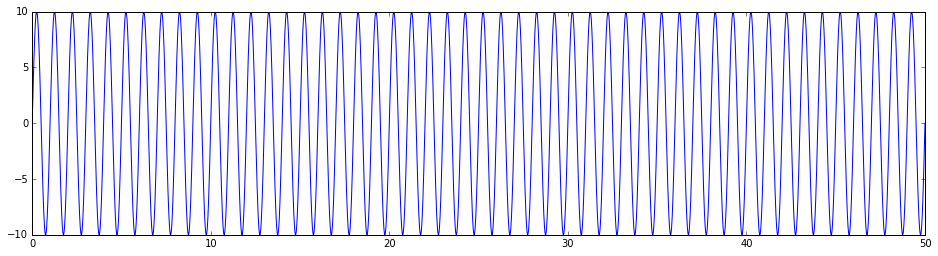In :
# next 50 "days"
t050to100 = np.arange(5001,10001)
syn050to100 = 20 + 10. * np.sin(t050to100 * (2*np.pi)/100.)
plt.figure(figsize=(16,4))
plt.plot(t050to100/100., syn050to100)

Out:
[<matplotlib.lines.Line2D at 0x1204154d0>]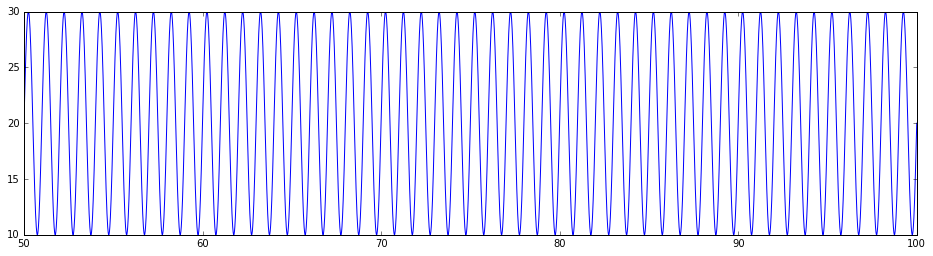In :
# next 50 "days"
t100to150 = np.arange(10001,15001)
syn100to150 = 20 + 10. * np.sin(t100to150 * (2*np.pi)/100.) + 20*np.sin(t100to150 * (2*np.pi)/5000.)
plt.figure(figsize=(16,4))
plt.plot(t100to150/100., syn100to150)

Out:
[<matplotlib.lines.Line2D at 0x1205f5110>]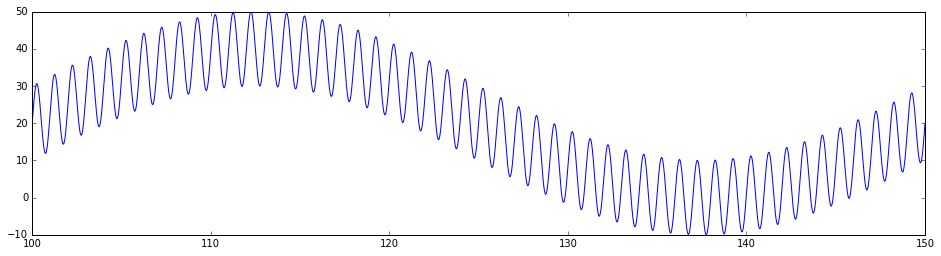In :
# next 50 "days"
t150to200 = np.arange(15001,20001)
syn150to200 = 20 + (10. * np.sin(t150to200 * (2*np.pi)/100.)) * (1*np.cos(t150to200 * (2*np.pi)/5000.))
plt.figure(figsize=(16,4))
plt.plot(t150to200/100., syn150to200)

Out:
[<matplotlib.lines.Line2D at 0x120877b90>]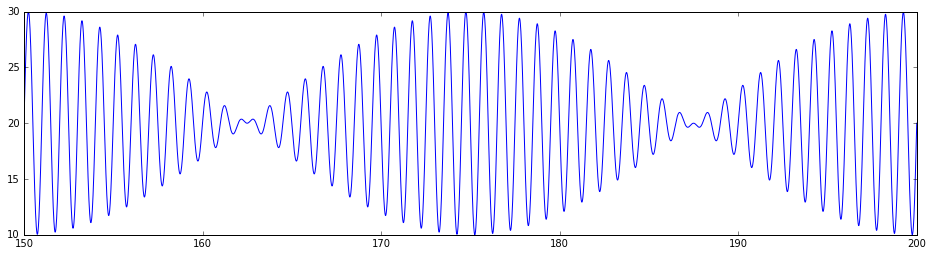In :
# next 50 "days"
t200to250 = np.arange(20001,25001)
syn200to250 = 20 + ((10. * np.sin(t200to250 * (2*np.pi)/100.)) * (1*np.cos(t200to250 * (2*np.pi)/5000.)) +
20*np.sin(t200to250 * (2*np.pi)/5000.) )
plt.figure(figsize=(16,4))
plt.plot(t200to250/100., syn200to250)

Out:
[<matplotlib.lines.Line2D at 0x120b3a750>]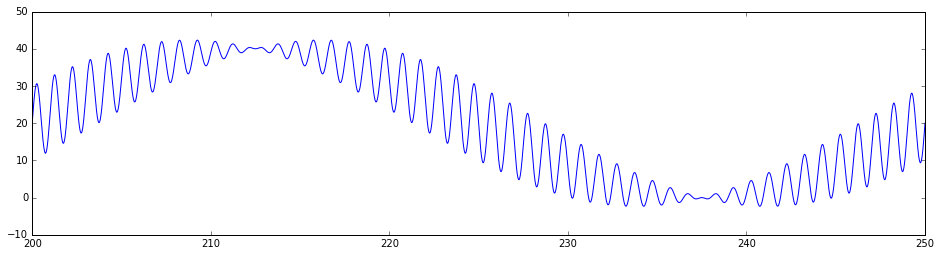In :
# next 50 "days"
t250to300 = np.arange(25001,30001)
np.random.seed(123456789)
syn250to300 = 20 + ((10. * np.sin(t250to300 * (2*np.pi)/100.)) * (1*np.cos(t250to300 * (2*np.pi)/5000.)) +
20*np.sin(t250to300 * (2*np.pi)/5000.) ) + 5 * np.random.randn(5000)
plt.figure(figsize=(16,4))
plt.plot(t250to300/100., syn250to300)

Out:
[<matplotlib.lines.Line2D at 0x120d891d0>]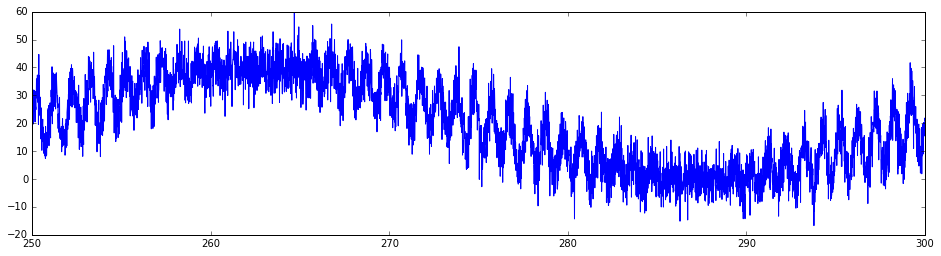In :
# all 300 "days
t000to300 = np.concatenate((t000to050,t050to100,t100to150,t150to200,t200to250, t250to300))
syn000to300 = np.concatenate((syn000to050,syn050to100,syn100to150,syn150to200,syn200to250, syn250to300))
plt.figure(figsize=(16,4))
plt.plot(t000to300/100., syn000to300)

Out:
[<matplotlib.lines.Line2D at 0x1212d9d90>]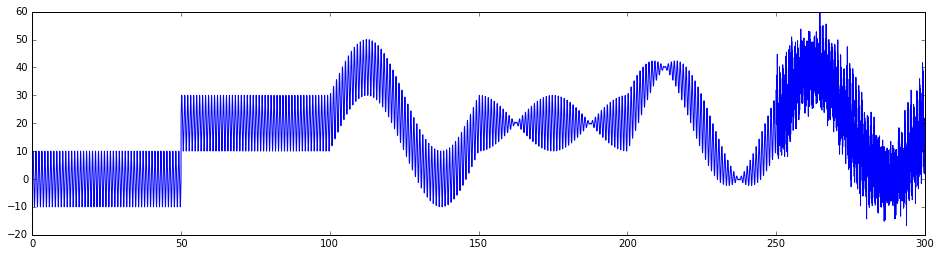## Apply SqDist Algorithm¶

In :
# set up Holt-Winters smoothing parameters
m = 100 # length of "day"
alpha = 1./100./3. # average age of level is 3 "days"
beta = 0 # slope doesn't change
gamma = 1./100.*100./3. # average age of "seasonal" correction is e "days"
phi = 1 # don't dampen the slope

# initialize states for HW smoother
l0 = None # this uses algorithm's default initial level
b0 = 0 # this is NOT the algorithm's default initial slope
s0 = None # this uses algorithm's default initial "seasonal" correction
sigma0 = [np.var(syn000to050)] # this is NOT the algorithm's default initial standard deviation


### First 50 "days"¶

Items to note:

• SQ+SV (green) gradually grows from zero to match the repeating signal;
• SV (red) has non-zero values because SQ has not yet adjusted to its full amplitude;
• SQ is adjusted by exponential smoothing with a lag of length m; HOWEVER,
• the SQ is also adjusted to enforce a zero-mean seasonal correction, which is why there is obvious non-zero SQ in the first m steps
In :
# first 50 "days"

# instantiate SqDistAlgorithm object
SqDist_syn = SqDistAlgorithm(m=m, alpha=alpha, beta=beta, gamma=gamma, phi=phi,
yhat0=None, s0=s0, l0=l0, b0=b0, sigma0=sigma0)

# define input stream
yobs_syn = Stream()
yobs_syn += Trace(syn000to050)

# process input stream
SvSqDistStream = SqDist_syn.process(yobs_syn)

plt.figure(figsize=(16,4))
plt.plot(t000to050/100., yobs_syn.data, color='blue')
plt.plot(t000to050/100., SvSqDistStream.data + SvSqDistStream.data, color='green')
plt.plot(t000to050/100., SvSqDistStream.data, color='red')

Out:
[<matplotlib.lines.Line2D at 0x120ab64d0>]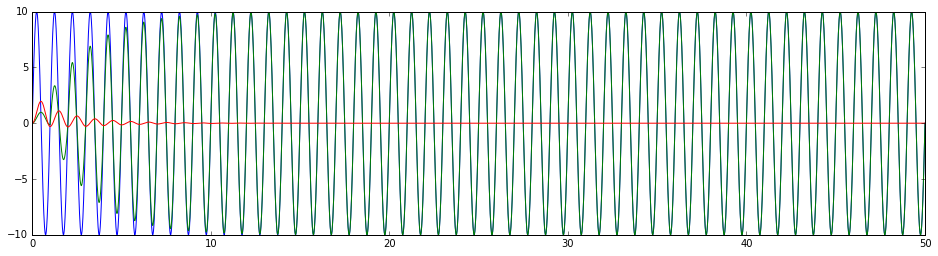### next 50 "days"¶

Items to note:

• SV does not adjust for a brief interval after the change in baseline level because the standard deviation of DIST had shrunk to a point where the new offset data points were treated as bad data; once the standard deviation of DIST increased to a critical level, new data were treated as valid, and the baseline level adjusted
• the "hiccup" in SQ is expected; it is ~1/3 the residual at the point the data became valid again; it gradually smooths out because it doesn't exist in the actual data
In :
# next 50 "days"

# define input stream
yobs_syn = Stream()
yobs_syn += Trace(syn050to100)
yobs_syn.stats.starttime = SqDist_syn.next_starttime

# process input stream
SvSqDistStream = SqDist_syn.process(yobs_syn)

plt.figure(figsize=(16,4))
plt.plot(t050to100/100., yobs_syn.data, color='blue')
plt.plot(t050to100/100., SvSqDistStream.data + SvSqDistStream.data, color='green')
plt.plot(t050to100/100., SvSqDistStream.data, color='red')

Out:
[<matplotlib.lines.Line2D at 0x121633550>]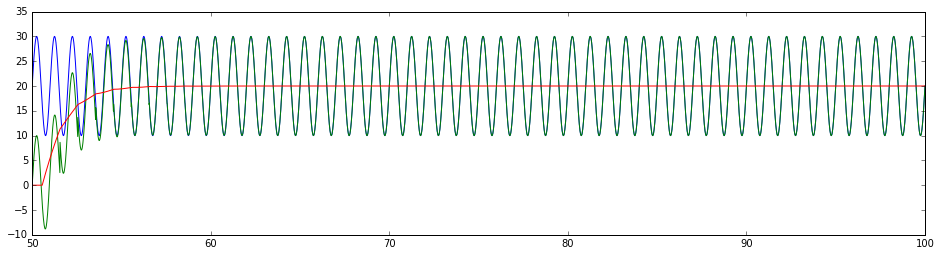### Next 50 "days"¶

Items to note:

• SV (red) tracks changing baseline level on which SQ variation is superimposed with a lag
• SQ (orange) remains more-or-less steady
In :
# next 50 "days"

# define input stream
yobs_syn = Stream()
yobs_syn += Trace(syn100to150)
yobs_syn.stats.starttime = SqDist_syn.next_starttime

# process input stream
SvSqDistStream = SqDist_syn.process(yobs_syn)

plt.figure(figsize=(16,4))
plt.plot(t100to150/100., yobs_syn.data, color='blue')
plt.plot(t100to150/100., SvSqDistStream.data + SvSqDistStream.data, color='green')
plt.plot(t100to150/100., SvSqDistStream.data, color='red')
plt.plot(t100to150/100., SvSqDistStream.data, color='orange')

Out:
[<matplotlib.lines.Line2D at 0x121ba0810>]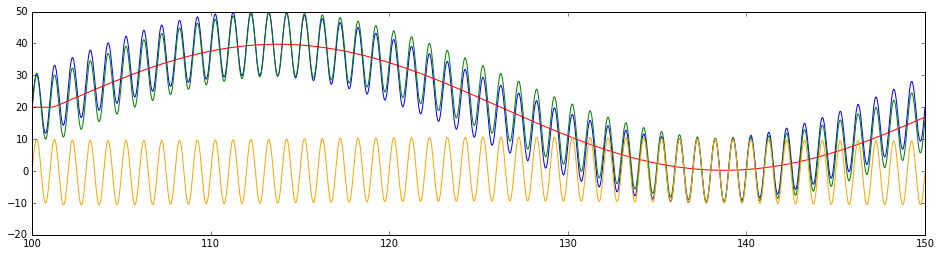### Next 50 "days"¶

Items to note:

• SQ+SV (green) tracks sine wave modulated signal with lag
• SV (red) is mostly the expected steady value, but erroneous oscillations tend to amplify when the relative amplitudes of SQ and the carrier signal differ substantially
In :
# next 50 "days"

# define input stream
yobs_syn = Stream()
yobs_syn += Trace(syn150to200)
yobs_syn.stats.starttime = SqDist_syn.next_starttime

# process input stream
SvSqDistStream = SqDist_syn.process(yobs_syn)

plt.figure(figsize=(16,4))
plt.plot(t150to200/100., yobs_syn.data, color='blue')
plt.plot(t150to200/100., SvSqDistStream.data + SvSqDistStream.data, color='green')
plt.plot(t150to200/100., SvSqDistStream.data, color='red')
plt.plot(t150to200/100., SvSqDistStream.data, color='orange')

Out:
[<matplotlib.lines.Line2D at 0x121eeefd0>]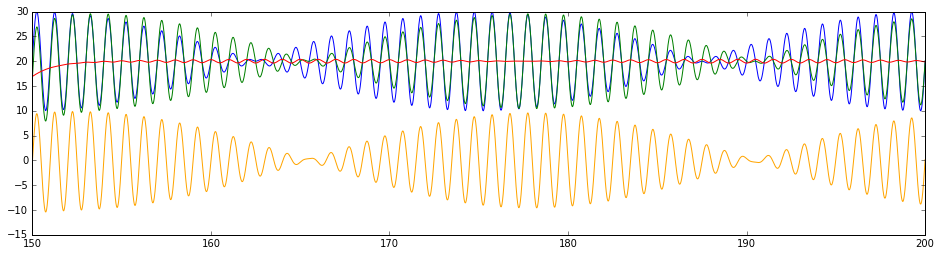### Next 50 "days"¶

Items to note:

• changing baseline level is tracked well by SV
In :
# next 50 "days"

# define input stream
yobs_syn = Stream()
yobs_syn += Trace(syn200to250)
yobs_syn.stats.starttime = SqDist_syn.next_starttime

# process input stream
SvSqDistStream = SqDist_syn.process(yobs_syn)

plt.figure(figsize=(16,4))
plt.plot(t200to250/100., yobs_syn.data, color='blue')
plt.plot(t200to250/100., SvSqDistStream.data + SvSqDistStream.data, color='green')
plt.plot(t200to250/100., SvSqDistStream.data, color='red')
plt.plot(t200to250/100., SvSqDistStream.data, color='orange')

Out:
[<matplotlib.lines.Line2D at 0x122257990>]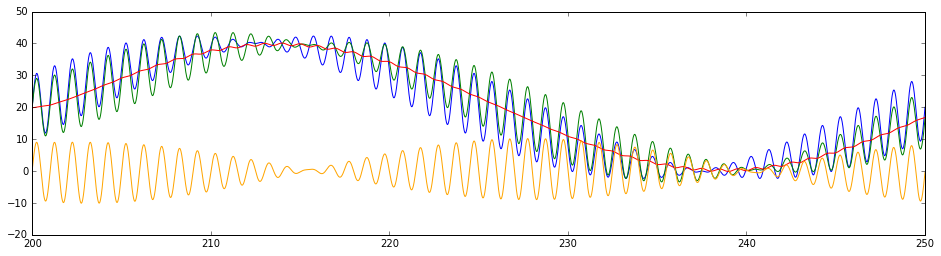### Next 50 "days"¶

Items to note:

• tracks reasonably well when signal-to-noise ratio is relatively large
In :
# next 50 "days"

# define input stream
yobs_syn = Stream()
yobs_syn += Trace(syn250to300)
yobs_syn.stats.starttime = SqDist_syn.next_starttime

# process input stream
SvSqDistStream = SqDist_syn.process(yobs_syn)

plt.figure(figsize=(16,4))
plt.plot(t250to300/100., yobs_syn.data, color='blue')
plt.plot(t250to300/100., SvSqDistStream.data + SvSqDistStream.data, color='green')
plt.plot(t250to300/100., SvSqDistStream.data, color='red')
plt.plot(t250to300/100., SvSqDistStream.data, color='orange')

Out:
[<matplotlib.lines.Line2D at 0x122065590>]